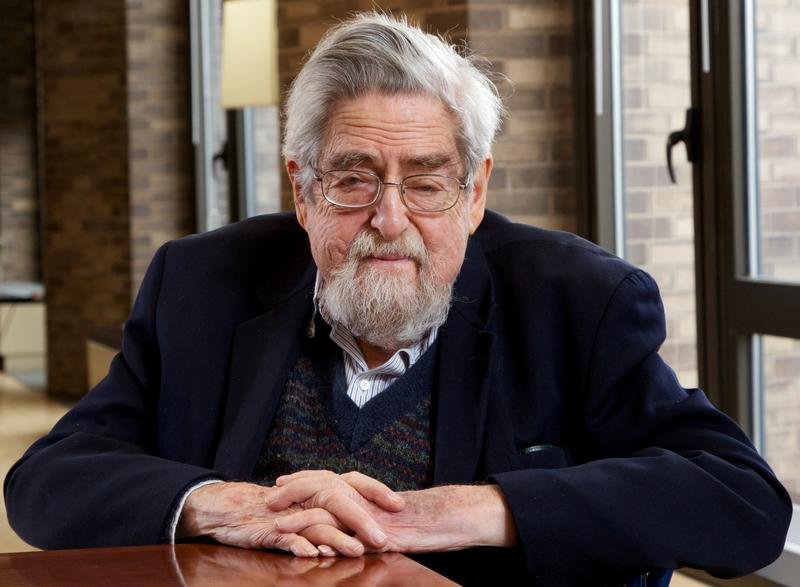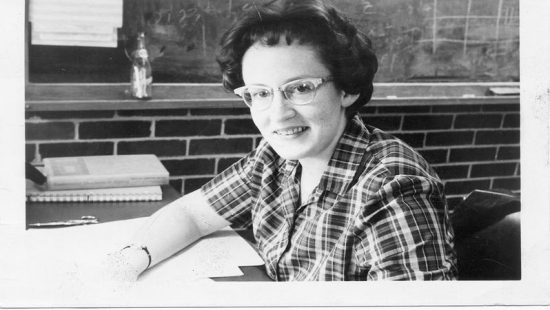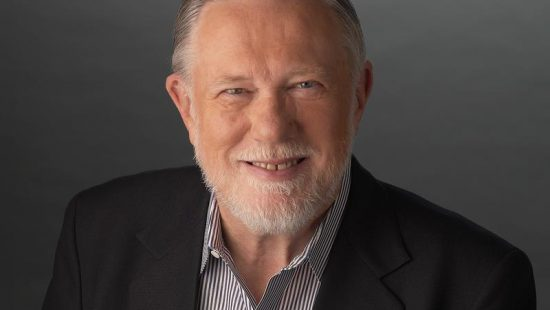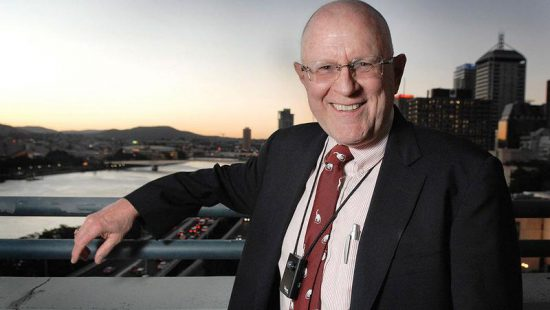# Louis Nirenberg

• National Medal of Science
• Computer Science

For his fundamental contributions to linear and nonlinear partial differential equations, and applications, particularly in geometry and complex analysis, thus having a decisive impact on the development of mathematics and its applications over a period of years.

In high school, Louis Nirenberg enjoyed physics, and thought he might become a physicist. “I didn’t even know that there was such a career as “mathematician.” I knew you could be a math teacher, but I didn’t know you could be a mathematician,” Nirenberg recounted in an interview. It was in college that Nirenberg discovered he could have a career in mathematics, and went on to make major contributions in the field.

Nirenberg made fundamental contributions to the understanding of linear and nonlinear partial differential equations, and their application to complex analysis and geometry. Nirenberg is considered by many as a world master of inequalities. For many years, mathematicians came from everywhere to seek his counsel on questions about inequalities.

His contributions include the Gagliardo–Nirenberg interpolation inequality, important in the solution of elliptic partial differential equations that are used in many areas of mathematics, and the formalization of the bounded mean oscillation, known as John–Nirenberg space, used to study the behavior of both elastic materials and games of chance.

By Jen Santisi

## Related Laureates### Mary Shaw### Charles Geschke